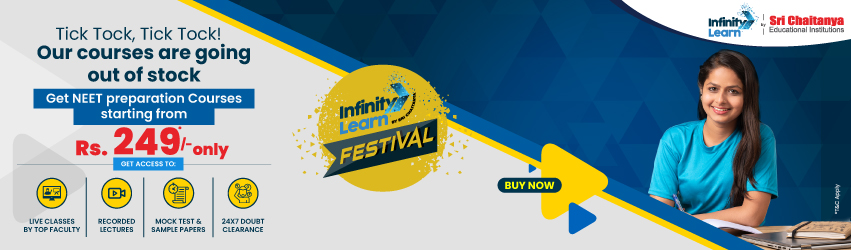RD-Sharma-class 9-maths-Solutions-chapter 8 – Lines and Angles -Exercise 8.3 Q 13

# RD-Sharma-class 9-maths-Solutions-chapter 8 – Lines and Angles -Exercise 8.3 Q 13Infinity Learn NEET Festival
Infinity Learn IIT JEE Festival

Get RD-Sharma-class 9-maths-Solutions-chapter 8 – Lines and Angles -Exercise 8.3 Q 13 for free on Infinity Learn. Infinity Learn provides free study materials and videos for CBSE Class 5 to 12 students.

RD-Sharma-class 9-maths-Solutions-chapter 8 – Lines and Angles -Exercise 8.3 Q 13 is very important study material for exam preparation. It helps students to score good marks in exams.

Need FREE NCERT/CBSE/IIT-JEE/NEET Study Material?

Infinity Learn provides detailed solutions to all the subjects chapter-wise.

Questions from RD-Sharma-class 9-maths-Solutions-chapter 8 – Lines and Angles -Exercise 8.3 Q 13 has been solved in the below images.

Get all the NCERT Solutions here

RD-Sharma-class 9-maths-Solutions-chapter 8 – Lines and Angles -Exercise 8.3 Q 13Join Infinity Learn Regular Class Programme!